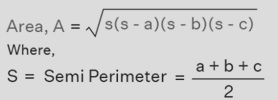# Rust program to calculate the area of a triangle given three sides

Given the sides of a triangle (a, b, and c), and calculate the area of a triangle given three sides using Rust program.
Submitted by Nidhi, on September 29, 2021

Problem Solution:

Here, we will read three sides of the triangle from the user. Then we will calculate the area of the triangle and print the result.

Formula to calculate the area of a triangle: The given sides are a, b, and c.Program/Source Code:

The source code to calculate the area of a triangle given three sides is given below. The given program is compiled and executed successfully.

```// Rust program to calculate the
// area of a triangle given three sides

use std::io;

fn main()
{
let mut a:f32 = 0.0;
let mut b:f32 = 0.0;
let mut c:f32 = 0.0;
let mut s:f32 = 0.0;
let mut area:f32 = 0.0;

let mut input1 = String::new();
let mut input2 = String::new();
let mut input3 = String::new();

println!("Enter first edge of triangle: ");
a = input1.trim().parse().expect("Not a valid number");

println!("Enter second edge of triangle: ");
b = input2.trim().parse().expect("Not a valid number");

println!("Enter third edge of triangle: ");
c = input3.trim().parse().expect("Not a valid number");

s = (a + b + c) / 2.0;
area = s * (s - a) * (s - b) * (s - c);
area = area.sqrt();

println!("Area of a triangle: {}", area);
}
```

Output:

```Enter first edge of triangle:
2
Enter second edge of triangle:
3
Enter third edge of triangle:
4
Area of a triangle: 2.9047375
```

Explanation:

Here, we read the three sides of the triangle from the user. Then we calculated the area of the triangle and printed the result.